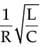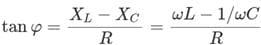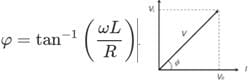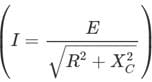# Assertion & Reason Test: Alternating Current - 2

## 15 Questions MCQ Test Physics Class 12 | Assertion & Reason Test: Alternating Current - 2

Description
Attempt Assertion & Reason Test: Alternating Current - 2 | 15 questions in 30 minutes | Mock test for NEET preparation | Free important questions MCQ to study Physics Class 12 for NEET Exam | Download free PDF with solutions
QUESTION: 1

### Directions : In the following questions, A statement of Assertion (A) is followed by a statement of Reason (R). Mark the correct choice as.Assertion (A) : Capacitor blocks dc and allows ac to pass.Reason (R): Capacitive reactance is inversely proportional to frequency.

Solution: Capacitive reactance = 1/2πfC

So, as f (frequency) increases, reactance decreases. For dc, frequency = 0, hence capacitor offers infinite reactance. So, it blocks dc. For ac, frequency ≠ 0,hence capacitor offers low reactance and allows ac to pass. Hence assertion and reason both are true. Assertion is properly explained by reason.

QUESTION: 2

### Directions : In the following questions, A statement of Assertion (A) is followed by a statement of Reason (R). Mark the correct choice as.Assertion (A): Both ac and dc can be measured by hot wire instrument.Reason (R): Hot wire instrument is based on the principal of magnetic effect of current.

Solution: In both ac and dc, heat generated is proportional to the square of current. Polarity change of ac is immaterial in the case of heat generation. Hence they can be measured by hot wire instrument. Hence, the assertion is true. Hot wire instruments are based on the principle of heating effect of current. Hence the reason is false.
QUESTION: 3

### Directions : In the following questions, A statement of Assertion (A) is followed by a statement of Reason (R). Mark the correct choice as.Assertion (A): At resonance, the current becomes minimum in a series LCR circuit.Reason (R): At resonance, voltage and current are out of phase in a series LCR circuit.

Solution: At resonance, XL = XC, so the circuit impedance becomes minimum and resistive and hence the current becomes maximum. So, the assertion is false. At resonance, XL = XC, so the circuit impedance becomes resistive. In resistive circuit voltage and current are always in same phase. Hence, reason is also false.
QUESTION: 4

Directions : In the following questions, A statement of Assertion (A) is followed by a statement of Reason (R). Mark the correct choice as.

Assertion (A): Quality factor of a series LCR circuit is Q =Reason (R): As bandwidth decreases, Q increases in a resonant LCR circuit.

Solution: Quality factor of a series LCR circuit is Q

=Quality factor is also defined as Q = Resonant frequency/Bandwidth. So, as bandwidth decreases, Q increases. So, reason is also true. But reason does not explain the assertion.

QUESTION: 5

Directions : In the following questions, A statement of Assertion (A) is followed by a statement of Reason (R). Mark the correct choice as.

Assertion (A): An alternator is a machine which converts mechanical energy into electrical energy.

Reason (R): When a coil rotates in a magnetic field an e.m.f. is induced in it.

Solution: Alternator is basically a generator in which a coil rotates in a strong magnetic field and according to laws of electromagnetic induction e.m.f. is generated. So, assertion and reason both are true and reason explains the assertion.
QUESTION: 6

Directions : In the following questions, A statement of Assertion (A) is followed by a statement of Reason (R). Mark the correct choice as.

Assertion (A): A step-up transformer converts input low AC voltage to output high AC voltage.

Reason (R): It violate the law of conservation of energy.

Solution: Step-up transformer means it converts input low AC voltage to output high AC voltage. So, the assertion is true. For step up transformer, VOUT / VIN >1, but simultaneously IOUT / IIN < 1 and PIN = POUT (ideally). Hence, the law of conservation of energy is not violated.
QUESTION: 7

Directions : In the following questions, A statement of Assertion (A) is followed by a statement of Reason (R). Mark the correct choice as.

Assertion: The alternating current lags behind the e.m.f. by a phase angle of π/2, when ac flows through an inductor.

Reason: The inductive reactance increases as the frequency of ac source decreases.

Solution: When ac flows through an inductor current lags behind the emf., by phase of p/2, inductive reactance, XL=ωL=π.2f.L, so when frequency increases correspondingly inductive reactance also increases.
QUESTION: 8

Directions : In the following questions, A statement of Assertion (A) is followed by a statement of Reason (R). Mark the correct choice as.

Assertion: When capacitive reactance is smaller than the inductive reactance in LCR current, e.m.f. leads the current .

Reason: The phase angle is the angle between the alternating e.m.f. and alternating current of the circuit.

Solution: The phase angle for the LCR circuit is given byWhere XL, XC are inductive reactance and capacitive reactance respectively when XL > XC then tanf is positive i.e. f is positive (between 0 and p/2). Hence emf leads the

QUESTION: 9

Directions : In the following questions, A statement of Assertion (A) is followed by a statement of Reason (R). Mark the correct choice as.

Assertion: If the frequency of alternating current in an ac circuit consisting of an inductance coil is increased then current gets decreased.

Reason: The current is inversely proportional to frequency of alternating current.

Solution: When frequency of alternating current is increased, the effective resistance of the inductive coil increases. Current (XL=ωL=2πfL) in the circuit containing inductor is given by I = V/XL = V/2πfL As inductive resistance of the inductor increases, current in the circuit decreases.
QUESTION: 10

Directions : In the following questions, A statement of Assertion (A) is followed by a statement of Reason (R). Mark the correct choice as.

Assertion: An alternating current does not show any magnetic effect.

Reason: Alternating current varies with time.

Solution: Like direct current, an alternating current also produces magnetic field. But the magnitude and direction of the field goes on changing continuously with time.
QUESTION: 11

Directions : In the following questions, A statement of Assertion (A) is followed by a statement of Reason (R). Mark the correct choice as.

Assertion: ac is more dangerous than dc

Reason: Frequency of ac is dangerous for human body.

Solution: The effect of ac on the body depends largely on the frequency. Low frequency currents of 50 to 60 Hz (cycles/sec), which are commonly used, are usually more dangerous than high frequency currents and are 3 to 5 times more dangerous than dc of same voltage and amperage (current). The usual frequency of 50 cps (or 60 cps) is extremely dangerous as it corresponds to the fibrillation frequency of the myocardium. This results in ventricular fibrillation and instant death.
QUESTION: 12

Directions : In the following questions, A statement of Assertion (A) is followed by a statement of Reason (R). Mark the correct choice as.

Assertion: The division are equally marked on the scale of ac ammeter.

Reason: Heat produced is directly proportional to the current.

Solution: An ac ammeter is constructed on the basics of heating effect of the electric current. Since heat produced varies as square of current (H=I2R). Therefore the division marked on the scale of ac ammeter are not equally spaced.
QUESTION: 13

Directions : In the following questions, A statement of Assertion (A) is followed by a statement of Reason (R). Mark the correct choice as.

Assertion: An inductance and a resistance are connected in series with an ac circuit. In this circuit the current and the potential difference across the resistance lag behind potential difference across the inductance by an angle p/2.

Reason: In LR circuit voltage leads the current by phase angle which depends on the value of inductance and resistance both.

Solution: As both the inductance and resistance are joined in series, hence current through both will be same. But in case of resistance, both the current and potential vary simultaneously, hence they are in same phase. In case of an inductance when current is zero, potential difference across it is maximum and when current reaches maximum (at w t = p/2), potential difference across it becomes zero i.e. potential difference leads the current by p/2 or current lags behind the potential difference by p/2, Phase angle in case of LR circuit is given asQUESTION: 14

Directions : In the following questions, A statement of Assertion (A) is followed by a statement of Reason (R). Mark the correct choice as.

Assertion: An electric lamp connected in series with a variable capacitor and ac source, its brightness increases with increase in capacitance.

Reason: Capacitive reactance decrease with increase in capacitance of capacitor.

Solution: Capacitive reactance XC = 1/ωC When capacitance increases, the capacitive reactance decreases. Due to decrease in its values, the current in the circuit will increasesand hence brightness of source (or electric lamp) will also increases.

QUESTION: 15

Directions : In the following questions, A statement of Assertion (A) is followed by a statement of Reason (R). Mark the correct choice as.

Assertion: Current through a pure inductor is wattless.

Reason: Phase difference between emf across a pure inductor and current through it is 90o or π/2

Solution: In a purely inductive circuit (an AC circuit containing inductance only) the current lags behind the voltage by π/2 .Use Code STAYHOME200 and get INR 200 additional OFF Use Coupon Code